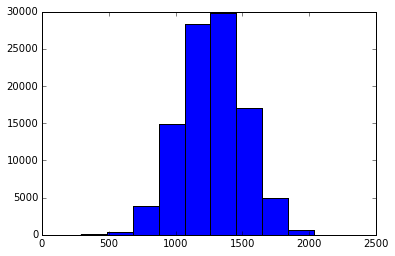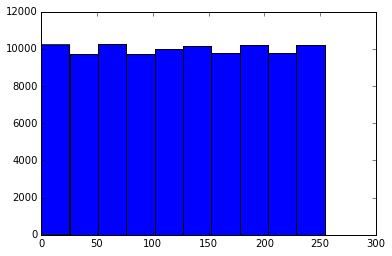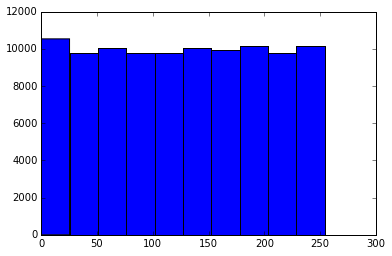In :
import math
import random
import matplotlib.pyplot as plt
import matplotlib
%matplotlib inline

In :
def random_sum(sampler, count):
return sum(sampler() for i in range(count))

def random_xor(sampler, count):
return reduce(lambda a,b: a^b, (sampler() for i in range(count)))

def sample_sum(samples):
return sum(samples)

def sample_xor(samples):
return reduce(lambda x, y: x^y, samples)

In :
count = 100000
n = 10
max_value = 2**8-1
sampler = lambda: random.randint(0, max_value)
samples =  [[sampler() for i in range(n)] for i in range(count)]
standard_samples = [sample_sum(sample) for sample in samples]
mod_samples = [sample_sum(sample) % max_value for sample in samples]
xor_samples = [sample_xor(sample) % max_value for sample in samples]

In :
plt.hist(standard_samples);The sum of independent random variables approaches a normal distribution due to the central limit theorem.

In :
plt.hist(mod_samples);The distribution of the modulo sum of random variables can become uniform again due to boundary conditions of a sort that are removed due to the modulo operation. See http://emmettmcquinn.com/blog/ for more.

In :
plt.hist(xor_samples);XOR is a common simple technique to combine entropy (see here for more).

In [ ]: